# SAT II Math II : Number Sets

## Example Questions

### Example Question #1 : Number Sets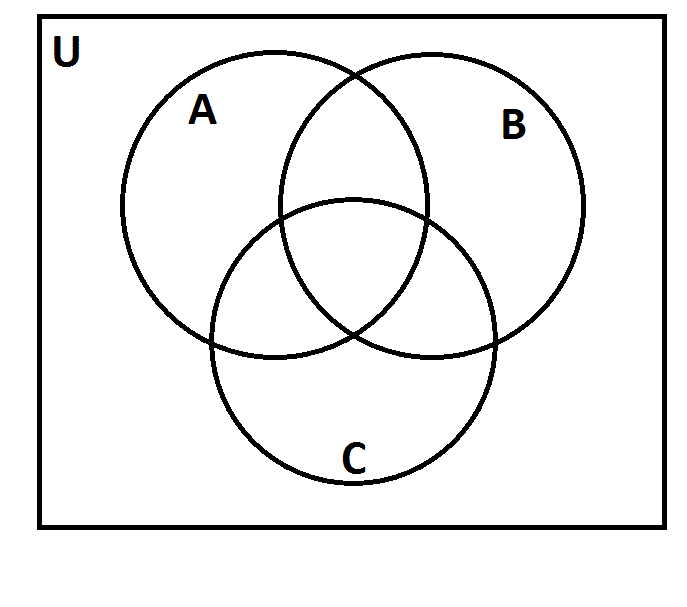The above represents a Venn diagram. The universal set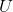is the set of all positive integers.

Letbe the set of all multiples of 2; letbe the set of all multiples of 3; letbe the set of all multiples of 5.

As you can see, the three sets divide the universal set into eight regions. Suppose each positive integer was placed in the correct region. Which of the following numbers would be in the same region as 873?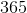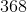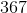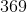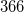Explanation:

From the last digit, it can be immediately determined that 873 is not a multple of 2 or 5; since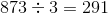, 873 is a multiple of 3. Therefore,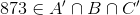We are looking for an integer that is also in this set - that is, one that is also a multiple of 3 but not 2 or 5. From the last digits, we can immediately eliminate 366 and 368 as multiples of 2 and 365 as a multiple of 5. We test 367 and 369 to see which one is a multiple of 3: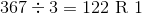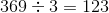369 is the correct choice.

### Example Question #2 : Number Sets

Which of the following sets is closed under multiplication?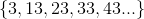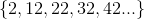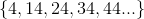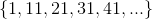Explanation:

A set is closed under multiplication if and only if the product of any two (not necessarily distinct) elements of that set is itself an element of that set.

This can easily be disproved in the case of three of these sets: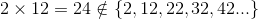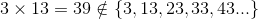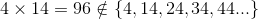But closure can be proved to hold in the case of. Each number takes the form of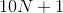for some nonnegative integer. If we multiply two numbers in this form, we get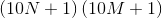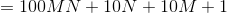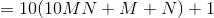which is an element of, being of this form.

### Example Question #3 : Number Sets

What is the power set for the data set?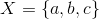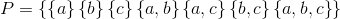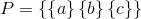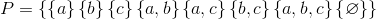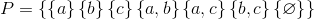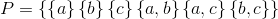Explanation:

The power set is the set of all subsets that can be created from the original set.

For the set, you can create the subsets: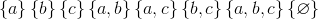This means that the power set is the set of all sets, so the power set is: